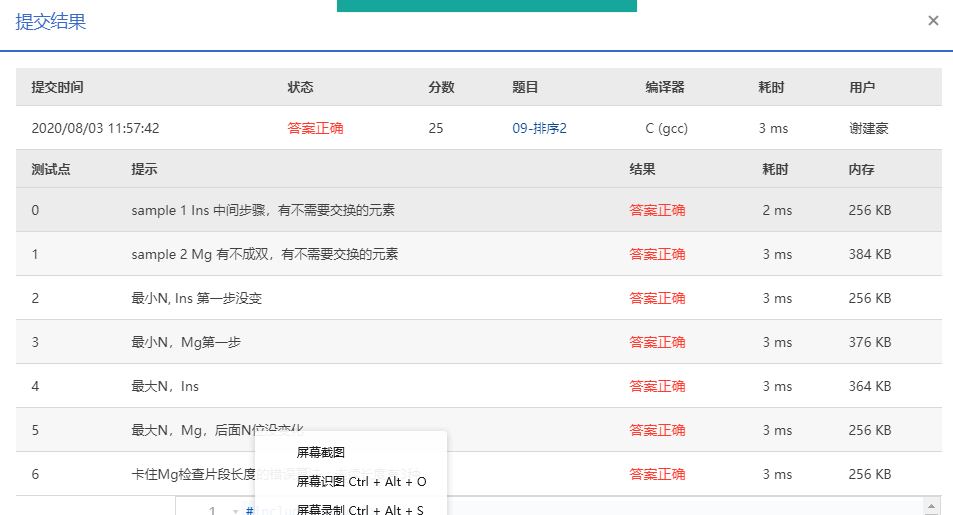# 09-排序2 Insert or Merge (25分) c语言实现

According to Wikipedia:

Insertion sort iterates, consuming one input element each repetition, and growing a sorted output list. Each iteration, insertion sort removes one element from the input data, finds the location it belongs within the sorted list, and inserts it there. It repeats until no input elements remain.

Merge sort works as follows: Divide the unsorted list into N sublists, each containing 1 element (a list of 1 element is considered sorted). Then repeatedly merge two adjacent sublists to produce new sorted sublists until there is only 1 sublist remaining.

Now given the initial sequence of integers, together with a sequence which is a result of several iterations of some sorting method, can you tell which sorting method we are using?

Input Specification:
Each input file contains one test case. For each case, the first line gives a positive integer N (≤100). Then in the next line, N integers are given as the initial sequence. The last line contains the partially sorted sequence of the N numbers. It is assumed that the target sequence is always ascending. All the numbers in a line are separated by a space.

Output Specification:
For each test case, print in the first line either “Insertion Sort” or “Merge Sort” to indicate the method used to obtain the partial result. Then run this method for one more iteration and output in the second line the resuling sequence. It is guaranteed that the answer is unique for each test case. All the numbers in a line must be separated by a space, and there must be no extra space at the end of the line.

Sample Input 1:
10
3 1 2 8 7 5 9 4 6 0
1 2 3 7 8 5 9 4 6 0
Sample Output 1:
Insertion Sort
1 2 3 5 7 8 9 4 6 0
Sample Input 2:
10
3 1 2 8 7 5 9 4 0 6
1 3 2 8 5 7 4 9 0 6
Sample Output 2:
Merge Sort
1 2 3 8 4 5 7 9 0 6

## 具体代码

#include<stdio.h>
#include<math.h>
#define MAX 100
int BIAO=0;
int B[MAX];
int BIJIAO(int n,int A[])
{

for(int i=0;i<n;i++)
if(A[i]!=B[i])
return 0;
return 1;
}
void Sort(int n,int A[],int B[])
{
int i,j;

int k;
int tep;
int sum=0;
int flag=0;
sum=BIJIAO(n,A);
for(i=1;i<n;i++)
{
tep=A[i];
for(j=i;j>0&&A[j-1]>tep;j--)
A[j]=A[j-1];
A[j]=tep;
if(sum==0)
sum=BIJIAO(n, A);
if(sum==1)
{
if(flag)
break;
flag=1;
}

}
if(sum!=1&&i==n)
sum=BIJIAO(n,A);

if(sum==1)
{
BIAO=1;

printf("Insertion Sort\n");
for(i=0;i<n;i++)
{
if(i!=0)
printf(" ");
printf("%d",A[i]);
}
}

}
void  Merge(int A[],int Tepa[],int L,int R,int RE)
{
int LE,NE,tep;
int i;
LE=R-1;
tep=L;
NE=RE-L+1;
while(L<=LE&&R<=RE)
if(A[L]<=A[R])
Tepa[tep++]=A[L++];
else
Tepa[tep++]=A[R++];
while(L<=LE)
Tepa[tep++]=A[L++];
while(R<=RE)
Tepa[tep++]=A[R++];
for(i=0;i<NE;i++,RE--)
A[RE]=Tepa[RE];
}
void Merge_pass( int A[], int TmpA[], int N, int length )
{ /* 两两归并相邻有序子列 */
int i, j;

for ( i=0; i <= N-2*length; i += 2*length )
Merge( A, TmpA, i, i+length, i+2*length-1 );
if ( i+length < N ) /* 归并最后2个子列*/
Merge( A, TmpA, i, i+length, N-1);
else /* 最后只剩1个子列*/
for ( j = i; j < N; j++ ) TmpA[j] = A[j];
}

void Merge_Sort( int A[], int N )
{
int length;
int *TmpA;
int flag=0;
int sum=0;
length = 1; /* 初始化子序列长度*/
TmpA = malloc( N * sizeof( int ) );
sum=BIJIAO(N,A);
if ( TmpA != NULL ) {
while( length < N ) {
Merge_pass( A, TmpA, N, length );
if(sum==0)
sum=BIJIAO(N,A);
length *= 2;
if(sum==1)
{
if(flag)
break;
flag=1;
}
Merge_pass( TmpA, A, N, length );
length *= 2;
if(sum==0)
sum=BIJIAO(N,TmpA);
if(sum==1)
{
if(flag)
break;
flag=1;
}
}
free( TmpA );
}
else printf( "空间不足" );
if(sum==1)
{

printf("Merge Sort\n");
for(int i=0;i<N;i++)
{
if(i!=0)
printf(" ");
printf("%d",A[i]);
}

}
}
int main()
{
int i,j;
int A[MAX],C[MAX];
scanf("%d",&j);
for(i=0;i<j;i++)
{
scanf("%d",&A[i]);
C[i]=A[i];
}
for(i=0;i<j;i++)
scanf("%d",&B[i]);

Sort(j,A,B);
if(BIAO==0)
Merge_Sort(C,j);

return 0;
}
/*6
1 3 2 6 5 4
1 2 3 5 6 4*/



## 具体实现05-0393
05-0567905-28337
08-2130
11-30514
05-1362
04-23102
07-1079
05-143389
07-27356